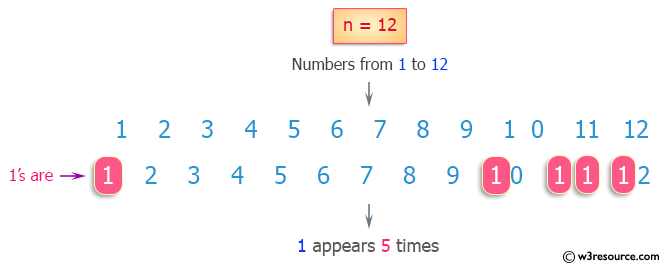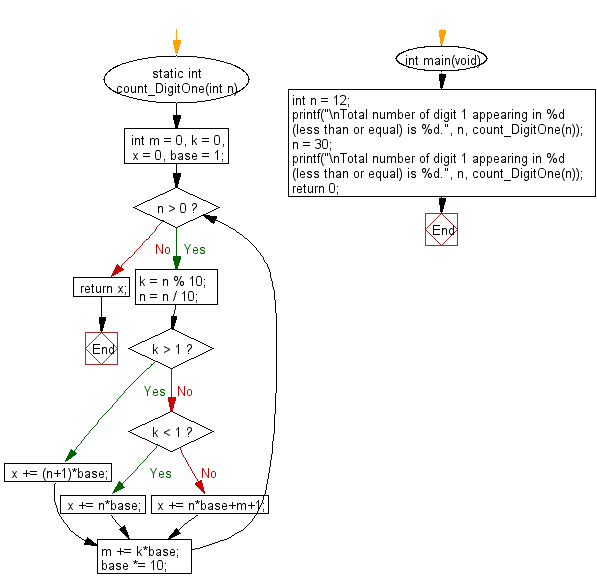﻿ C Program: Integers contain 1 less than or equal to n

# C Exercises: Count the total number of digit 1 appearing in all positive integers less than or equal to a given integer n

## C Programming Mathematics: Exercise-11 with Solution

Write a C program to count the total number of digits 1 appearing in all positive integers less than or equal to a given integer n.

For example:
Input n = 12,
Return 5, because digit 1 occurred 5 times in the following numbers: 1, 10, 11, 12.

Example:
Input:
n = 12
n = 30
Output:
Total number of digit 1 appearing in 12 (less than or equal) is 5.
Total number of digit 1 appearing in 30 (less than or equal) is 13.

Pictorial Presentation:Sample Solution:

C Code:

``````#include <stdio.h>

static int count_DigitOne(int n) {
int m = 0, k = 0, x = 0, base = 1;
while (n > 0) {
k = n % 10;
n = n / 10;

if (k > 1) { x += (n+1)*base; }
else if (k < 1) { x += n*base; }
else { x += n*base+m+1; }

m += k*base;
base *= 10;
}
return x;
}

int main(void)
{
int n = 12;
printf("\nTotal number of digit 1 appearing in %d (less than or equal) is %d.", n, count_DigitOne(n));
n = 30;
printf("\nTotal number of digit 1 appearing in %d (less than or equal) is %d.", n, count_DigitOne(n));
return 0;
}
```
```

Sample Output:

```Total number of digit 1 appearing in 12 (less than or equal) is 5.
Total number of digit 1 appearing in 30 (less than or equal) is 13.
```

Flowchart:C Programming Code Editor:

Improve this sample solution and post your code through Disqus.

What is the difficulty level of this exercise?

Test your Programming skills with w3resource's quiz.

﻿